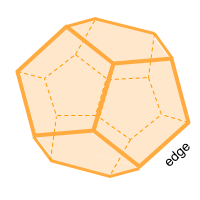Home » Polyhedra » Platonic Solids » Dodecahedron

# Volume of a DodecahedronVolume =
15 + (7 × √5) /4
× Edge ³

## Volume calculator for a dodecahedron

Enter the length of the edge of the dodecahedron to get the volume automatically.

## Description, how many faces, edges and vertices are there in a dodecahedron

The dodecahedron, (dodeca = 12 and Hedron = Face) is a solid of 12 faces where each of these faces is an equal regular pentagon, it has 30 edges and 20 vertices. At each of the vertices, 3 edges meet.

## Examples of a dodecahedron

We can find many objects that look like an dodecahedron, examples of dodecahedron shaped objects are: 12-sided dice used in some board games. Can you think of any other examples? leave a comment in the comment section at the bottom of the page.

## Formula for the volume of a dodecahedron

To calculate the volume of a dodecahedron you can calculate the volume of 12 equal pentagonal pyramids joint by their vertex. The difficulty is being able to calculate the height of these pyramids, but we can make use of the following formula, where we only need the value of an edge. You can also use the online calculator to calculate the volume of the dodecahedron automatically.

Volume =
15 + (7 × √5) /4
× Edge ³

Share